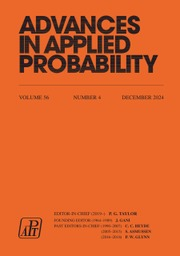Home
Hostname: page-component-cf9d5c678-r9vz2 Total loading time: 0.214 Render date: 2021-07-28T07:32:18.117Z Has data issue: true Feature Flags: { "shouldUseShareProductTool": true, "shouldUseHypothesis": true, "isUnsiloEnabled": true, "metricsAbstractViews": false, "figures": true, "newCiteModal": false, "newCitedByModal": true, "newEcommerce": true, "newUsageEvents": true }Advances in Applied Probability

# Projective Stochastic Equations and Nonlinear Long Memory

Published online by Cambridge University Press:  22 February 2016

## Abstract

A projective moving average {X t , t ∈ ℤ} is a Bernoulli shift written as a backward martingale transform of the innovation sequence. We introduce a new class of nonlinear stochastic equations for projective moving averages, termed projective equations, involving a (nonlinear) kernel Q and a linear combination of projections of X t on ‘intermediate’ lagged innovation subspaces with given coefficients α i and β i,j . The class of such equations includes usual moving average processes and the Volterra series of the LARCH model. Solvability of projective equations is obtained using a recursive equality for projections of the solution X t . We show that, under certain conditions on Q, α i , and β i,j , this solution exhibits covariance and distributional long memory, with fractional Brownian motion as the limit of the corresponding partial sums process.

## MSC classification

Type
General Applied Probability
Information
Advances in Applied Probability , December 2014 , pp. 1084 - 1105

## References

Baillie, R. T. and Kapetanios, G. (2008). Nonlinear models for strongly dependent processes with financial applications. J. Econometrics 147, 6071.CrossRefGoogle Scholar
Beran, J. (1994). Statistics for Long-Memory Processes (Monogr. Statist. Appl. Prob. 61). Chapman and Hall, New York.Google Scholar
Berkes, I. and Horváth, L. (2003). Asymptotic results for long memory LARCH sequences. Ann. Appl. Prob. 13, 641668.Google Scholar
Davydov, Yu. A. (1970). The invariance principle for stationary process. Theory Prob. Appl. 15, 487498.CrossRefGoogle Scholar
Dedecker, J. and Merlevède, F. (2003). The conditional central limit theorem in Hilbert spaces. Stoch. Process. Appl. 108, 229262.CrossRefGoogle Scholar
Dedecker, J. et al. (2007). Weak Dependence (Lecture Notes Statist. 190). Springer, New York.CrossRefGoogle Scholar
Doukhan, P., Lang, G. and Surgailis, D. (2012). A class of Bernoulli shifts with long memory: asymptotics of the partial sums process. Preprint. University of Cergy-Pontoise.Google Scholar
Doukhan, P., Oppenheim, G. and Taqqu, M. (eds) (2003). Theory and Applications of Long-Range Dependence. Birkhäuser, Boston, MA.Google Scholar
Giraitis, L. and Surgailis, D. (2002). ARCH-type bilinear models with double long memory. Stoch. Process. Appl. 100, 275300.CrossRefGoogle Scholar
Giraitis, L., Koul, H. L. and Surgailis, D. (2012). Large Sample Inference for Long Memory Processes. Imperial College Press, London.CrossRefGoogle Scholar
Giraitis, L., Leipus, R. and Surgailis, D. (2009). ARCH(∞) models and long memory properties. In Handbook of Financial Time Series, eds Mikosch, T. et al., Springer, Berlin, pp. 7184.CrossRefGoogle Scholar
Giraitis, L., Robinson, P. M. and Surgailis, D. (2000). A model for long memory conditional heteroskedasticity. Ann. Appl. Prob. 10, 10021024.Google Scholar
Giraitis, L., Leipus, R., Robinson, P. M. and Surgailis, D. (2004). LARCH, leverage, and long memory. J. Financial Econometrics 2, 177210.CrossRefGoogle Scholar
Hall, P. and Heyde, C. C. (1980). Martingale Limit Theory and Application. Academic Press, New York.Google Scholar
Hitczenko, P. (1990). Best constants in martingale version of Rosenthal's inequality. Ann. Prob. 18, 16561668.CrossRefGoogle Scholar
Ho, H.-C. and Hsing, T. (1997). Limit theorems for functionals of moving averages. Ann. Prob. 25, 16361669.CrossRefGoogle Scholar
Philippe, A., Surgailis, D. and Viano, M.-C. (2006). Invariance principle for a class of non stationary processes with long memory. C. R. Math. Acad. Sci. Paris 342, 269274.CrossRefGoogle Scholar
Philippe, A., Surgailis, D. and Viano, M.-C. (2008). Time-varying fractionally integrated processes with nonstationary long memory. Theory Prob. Appl. 52, 651673.CrossRefGoogle Scholar
Robinson, P. M. (1991). Testing for strong serial correlation and dynamic conditional heteroskedasticity in multiple regression. J. Econometrics 47, 6784.CrossRefGoogle Scholar
Robinson, P. M. (2001). The memory of stochastic volatility models. J. Econometrics 101, 195218.CrossRefGoogle Scholar
Stout, W. F. (1974). Almost Sure Convergence. Academic Press, New York.Google Scholar
Taqqu, M. S. (1979). Convergence of integrated processes of arbitrary Hermite rank. Z. Wahrscheinlichkeitsth. 50, 5383.CrossRefGoogle Scholar
Wu, W. B. (2005). Nonlinear system theory: another look at dependence. Proc. Nat. Acad. Sci. USA 102, 1415014154.CrossRefGoogle Scholar
Wu, W. B. and Min, W. (2005). On linear processes with dependent innovations. Stoch. Process. Appl. 115, 939958.Google Scholar
Wu, W. B. and Shao, X. (2006). Invariance principles for fractionally integrated nonlinear processes. In Recent Developments in Nonparametric Inference and Probability (IMS Lecture Notes Monogr. Ser. 50), Institute of Mathematical Statistics, Beachwood, OH, pp. 2030.CrossRefGoogle ScholarYou have Access
1
Cited by

# Send article to Kindle

Note you can select to send to either the @free.kindle.com or @kindle.com variations. ‘@free.kindle.com’ emails are free but can only be sent to your device when it is connected to wi-fi. ‘@kindle.com’ emails can be delivered even when you are not connected to wi-fi, but note that service fees apply.

Find out more about the Kindle Personal Document Service.

Projective Stochastic Equations and Nonlinear Long Memory
Available formats
×

# Send article to Dropbox

To send this article to your Dropbox account, please select one or more formats and confirm that you agree to abide by our usage policies. If this is the first time you use this feature, you will be asked to authorise Cambridge Core to connect with your <service> account. Find out more about sending content to Dropbox.

Projective Stochastic Equations and Nonlinear Long Memory
Available formats
×

# Send article to Google Drive

To send this article to your Google Drive account, please select one or more formats and confirm that you agree to abide by our usage policies. If this is the first time you use this feature, you will be asked to authorise Cambridge Core to connect with your <service> account. Find out more about sending content to Google Drive.

Projective Stochastic Equations and Nonlinear Long Memory
Available formats
×
×